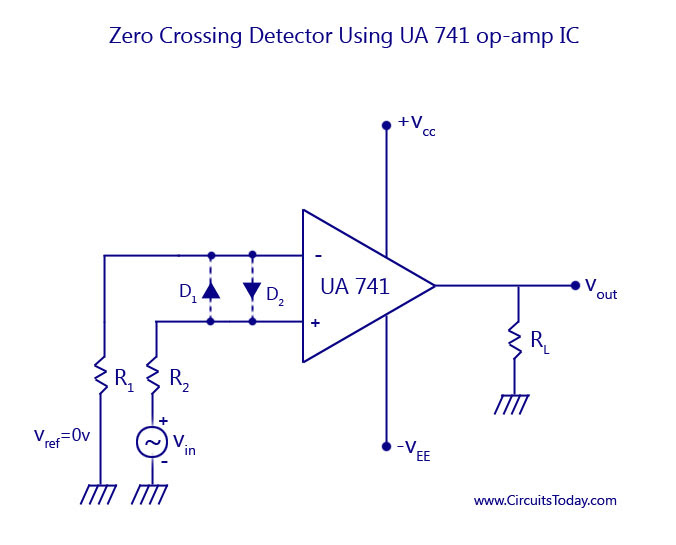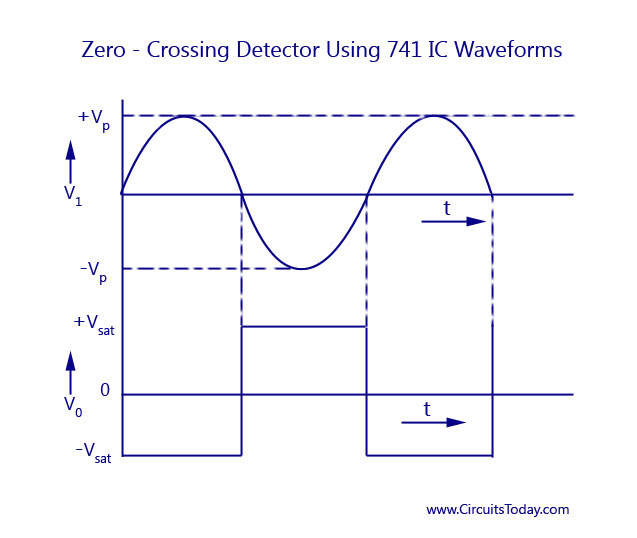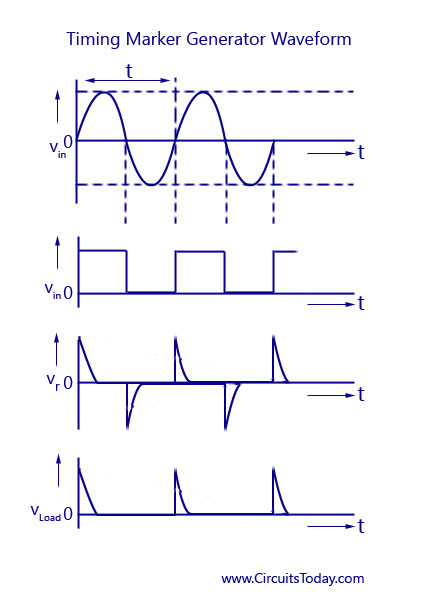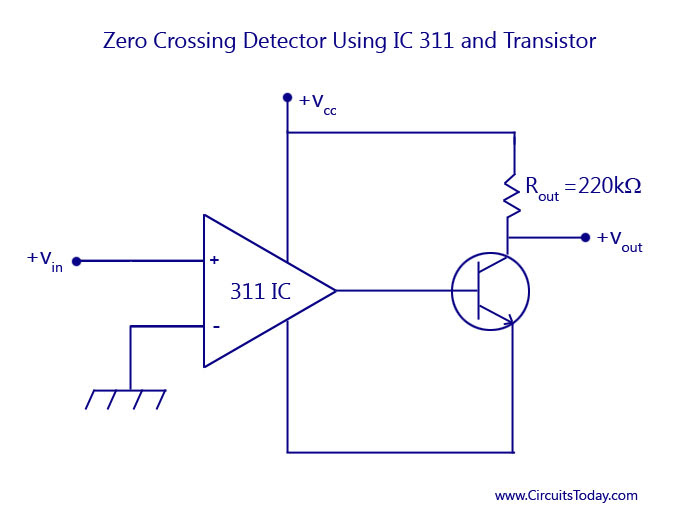## Audio

[Audio][slideshow]

In this article, we discuss Zero Crossing Detector in detail with two different circuits. In the initial paragraphs of the tutorial, you will learn zero crossing detector using op amp designed using the popular 741 IC. We have a neat draw circuit diagram of zero crossing detector and we have explained its working principle and theory behind the scene in easy to understand words. Towards the middle of this tutorial, you will learn about 2 applications of zero crossing detector – which are time marker generator and phasemeter. Towards the end of article, we have drawn another circuit diagram of zero crossing detector designed using IC 311 and transistor.

### Zero Crossing Detector using 741 IC

The zero crossing detector circuit is an important application of the op-amp comparator circuit. It can also be called as the sine to square wave converter. Anyone of the inverting or non-inverting comparators can be used as a zero-crossing detector. The only change to be brought in is the reference voltage with which the input voltage is to be compared, must be made zero (Vref = 0V). An input sine wave is given as Vin. These are shown in the circuit diagram and input and output waveforms of an inverting comparator with a 0V reference voltage.Zero-Crossing Detector Using UA741 op-amp IC

As shown in the waveform, for a reference voltage 0V, when the input sine wave passes through zero and goes in positive direction, the output voltage Vout is driven into negative saturation. Similarly, when the input voltage passes through zero and goes in the negative direction, the output voltage is driven to positive saturation. The diodes D1 and D2 are also called clamp diodes. They are used to protect the op-amp from damage due to increase in input voltage. They clamp the differential input voltages to either +0.7V or -0.7V.

In certain applications, the input voltage may be a low frequency waveform. This means that the waveform only changes slowly. This causes a delay in time for the input voltage to cross the zero-level. This causes further delay for the output voltage to switch between the upper and lower saturation levels. At the same time, the input noises in the op-amp may cause the output voltage to switch between the saturation levels. Thus zero crossing are detected for noise voltages in addition to the input voltage. These difficulties can be removed by using a regenerative feedback circuit with a positive feedback that causes the output voltage to change faster thereby eliminating the possibility of any false zero crossing due to noise voltages at the op-amp input.Zero-Crossing Detector Using 741IC -Waveforms

### Zero-crossing Detector as Time Marker Generator

For an input sine wave, the output of the zero-crossing detector being a square wave, is further passed through an RC series circuit. This is shown in the figure below.Zero-Crossing Detector Using Timing Marker Generator

If the time constant RC is very small compared to the period T of the input sine wave, then the voltage across R of the RC circuit network called Vr will be a series of positive and negative pulses. If the voltage Vr is applied to a clipper circuit using a diode D, the load voltage Vload will have only positive pulses and will clip away the negative pulses. Thus, a zero-crossing detector whose input is a sign wave has been converted into a train of positive pulses at interval T by adding a RC network and a clipping circuit.Time Marker Generator Waveform

### Zero-crossing Detector as Phasemeter

A zero-crossing detector can be used for the measurement of phase angle between two voltages. The working will be the same as explained in the above circuit. A train of pulses in the positive and negative cycles are obtained and the time interval between the pulse of sine wave voltage and that of second sine wave voltage is measured. This interval of time is proportional to the phase difference between the two input sine wave voltages. The range of use of phasemeter for measurement is 0° to 360°.

### Zero-crossing Detector Using IC 311 and Transistor

A zero-crossing detector using an 8 pin DIP unit 311 IC is shown in the figure below. The output of the 311 op-amp IC is connected to an open collector NPN transistor. A 20 kiloohm load resistor is connected to the output of the transistor.Zero-Crossing Detector Using IC 311 and Transistor

For a positive input signal, that is, for an input sine wave above 0 volt, the output of the op-amp sets the transistor OFF, and the output of the transistor goes HIGH. The low condition resembles a -10 volt in the circuit and a HIGH condition resembles -10 volt in the circuit.

The output of the transistor indicates whether the input is more than or less than 0 volt. In short, if the input signal is a positive voltage, the output of the transistor will be LOW. If the input signal is a negative voltage, the output of the transistor will be HIGH.

[Lights][grids]